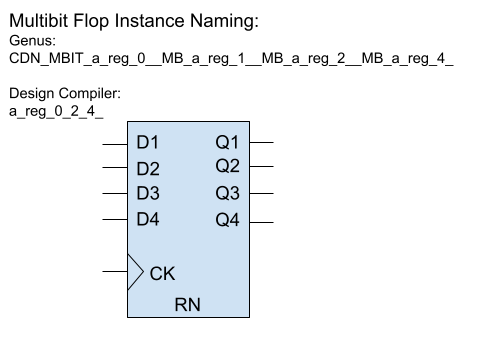﻿ NanDigits: Netlist Processing Platform GOF ECO GOF Formal

## Multibit flops in ECO

Multibit flops can pose a challenge in logic equivalence check and ECO due to the different naming conventions used by various synthesis tools. For example, as depicted in Figure 1, a four-bit multibit flop has a different naming style in Cadence Genus compared to Synopsys Design Compiler after name changing. Additionally, backend tools may split some multibit flops into single bit flops to address timing issues. These factors make key point mapping a complex task.

In logic equivalence check, multibit flops need to be mapped to single flops. However, the mapping of single flops to multibit flops from the Reference Netlist may differ from the Implementation Netlist. For instance, in Figure 1, the Implementation Netlist has a four-bit multibit flop instance named 'a_reg_0_2_4_', whereas the Reference Netlist after Synthesis may have two-bit multibit flops named 'a_reg_0_1_' and 'a_reg_2_4_'. Depending solely on naming conventions may not lead to the correct multibit to single bit mapping. Although LEC and ECO tools can handle some limited multibit to single bit mapping using comprehensive algorithms, there is no guarantee of complete successful mapping.Figure 1: Multibit flop naming in synthesis tools

GOF provides support for accurate and reliable key point mapping through the use of text mode SVF files from Design Compiler. These SVF files are encrypted by default, but can be converted to text mode when using Formality to read the encrypted file. Additionally, GOF can convert backend multibit flop split/merge information into an SVF file. By reading both the synthesis SVF and the converted SVF file, GOF is able to completely resolve the mapping of multibit flops to single bit flops.

For instance, Innovus generates a multi_bit_pin_mapping file to store split and merge information. This file can be converted to an SVF text file using a GOF script.

Here is an example script for converting an Innovus multi_bit_pin_mapping file:

```read_library("libdir/art.lib");
set_multibit_blasting(0); # Disable multibit blasting
set_top("the_top");

open(FIN, "./multi_bit_pin_mapping");
my \$mbit_split = {};
my \$mbit_merge = {};
while(){
my (\$from, \$to) = (m/(\S+)\s+(\S+)/);
\$from =~ s/\/\w+\$//; # remove the pin
\$to =~ s/\/\w+\$//;
my (\$module, \$to_inst) = get_resolved(\$to);
my (\$from_inst) = (\$from =~ m/([^\/]+)\$/);
my \$libcell = get_ref(\$to);
gprint("get ref of \$to as \$libcell\n");
my \$is_ff = is_seq(\$libcell, "-ff");
if(\$is_ff){
if(is_seq(\$libcell, "-bank")==0){
if(!exists \$mbit_split->{\$module}{\$from_inst}){
\$mbit_split->{\$module}{\$from_inst} = [];
}
if(grep(\$_ eq \$to_inst, @{\$mbit_split->{\$module}{\$from_inst}})==0){
gprint("Multibit split in \$module \$from_inst to \$to_inst\n");
push @{\$mbit_split->{\$module}{\$from_inst}}, \$to_inst;
}
}else{
# Bank
if(!exists \$mbit_merge->{\$module}{\$to_inst}){
\$mbit_merge->{\$module}{\$to_inst} = [];
}
if(grep(\$_ eq \$from_inst, @{\$mbit_merge->{\$module}{\$to_inst}})==0){
gprint("Multibit merge in \$module \$from_inst to \$to_inst\n");
push @{\$mbit_merge->{\$module}{\$to_inst}}, \$from_inst;
}
}
}
}
close(FIN);

my \$svf = "";
foreach my \$module (keys %\$mbit_merge){
\$svf .= "guide_multibit -design \$module -type { svfMultibitTypeBank } \\\n";
\$svf .= "  -groups { \\\n";
foreach my \$mbit_inst (keys %{\$mbit_merge->{\$module}}){
my \$i_st = "";
my \$cnt = 0;
foreach my \$s_bit (@{\$mbit_merge->{\$module}{\$mbit_inst}}){
\$i_st .= " \$s_bit 1";
\$cnt++;
}
\$i_st .= " \$mbit_inst \$cnt";
\$svf .= "\t{ \$i_st } \\\n";
}
\$svf .= "    }\n";
}
foreach my \$module (keys %\$mbit_split){
\$svf .= "guide_multibit -design \$module -type { svfMultibitTypeSplit } \\\n";
\$svf .= "  -groups { \\\n";
foreach my \$mbit_inst (keys %{\$mbit_split->{\$module}}){
my \$i_st = "";
my \$cnt = 0;
foreach my \$s_bit (@{\$mbit_split->{\$module}{\$mbit_inst}}){
\$i_st .= " \$s_bit 1";
\$cnt++;
}
\$i_st = " \$mbit_inst \$cnt \$i_st";
\$svf .= "\t{ \$i_st } \\\n";
}
\$svf .= "    }\n";
}
open(FOUT, ">backend_multibit.svf.txt");
print FOUT \$svf;
close(FOUT);
```

```read_svf("-ref", "reference.svf.txt");
Follow us: